Physic Tutorials

# Solutions to some physics UTME past questions

Question 1

A bullet fired vertically upward from a gun held 2.0m above the ground reaches its maximum height in 4.0 s. calculate its initial velocity. {2009}

A.10ms-1           B.8ms-1                              C. 40ms-1                                                  D. 20ms-1

[g = 10ms-2]

Solution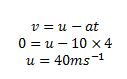C is the correct option

Question 2

An object of mass 80kg is pulled on a horizontal rough ground by a force of 500N. Find the coefficient of static friction. {2009}

A.0.8                   B. 0.4                              C. 1.0                           D. 0.6

[g = 10ms-2]

SolutionD is the correct option

Question 3

Two cars moving in the same direction have speeds of 100kmh-1 and 130kmh-1. What is the velocity of the faster car as measured by an observer in the slower car? {2010}

A.130kmh-1                      B. 230kmh-1                   C. 200kmh-1                                            D. 30kmh-1

Solution

You are to calculate the relative speedD is the correct option

Question 4

A car moves with an initial velocity of 25ms-1 and reaches a velocity of 45ms-1 in 10s. What is the acceleration of the car?{2010}

1. 5ms-1 B. 25ms-1 C. 20ms-1                                                 D. 2ms-1

SolutionD is the correct option

Question 5

Two balls X and Y weighing 5g and 50kg respectively were thrown up vertically at the same time with a velocity of 100ms-1. How will their positions be one second later? {2011}

A. X and Y will both be 500m from the point of throw

B. X and Y will be 500m from each other

C. Y will be 500 m ahead of X

D. X will be 500m ahead of Y.

Solution

The distance covered after one second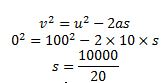S = 500m

The position the two balls will be from the point of throw is independent on their mass.

A is the correct option

Question 6

If it takes an object 3s to fall freely to the ground from a certain height, what is the distance covered by the object?

A. 60 m       B. 90 m      C. 30 m                             D. 45 m.

[g = 10ms-2]

Solution

Initial velocity = 0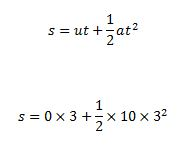S = 45m

D is the correct option

Question 7

Calculate the total distance covered by a train before coming to rest if its initial speed is 30ms-1 with a constant retardation of 0.1ms-2. {2012}

A. 5500m

B. 4500m

C. 4200m

D. 3000m.

Solution

V = 0

U =30ms-1

a=-0.1ms-2

The a is negative because the motion is retarding i.e. deceleration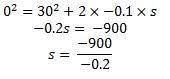S = 4500 m

B is the correct option

Question 8

A car starts from rest and moves with a uniform acceleration of 30ms-2 for 20s. Calculate the distance covered at the end of the motion.{2012}

A. 6km

B. 12km

C. 18km

D. 24km.

Solution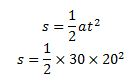S = 6000m = 6km

A is the correct option

Question 9

An object of mass 20kg slides down an inclined plane at an angle of 30o to the horizontal. The coefficient of static friction is  {2012}

A. 0.2            B. 0.3             C. 0.5                            D. 0.6

[g = 10ms-2]

SolutionQuestion 10

A train with an initial velocity of 20ms-1 is subjected to a uniform deceleration of 2ms-2. The time required to bring the train to a complete halt is

1. 40s B. 5s                          C. 10s                                D. 20s

Solution

V = 0

U = 20

a= -2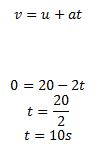C is the correct option

Question 11

Calculate the apparent weight loss of a man weighing 70kg in an elevator moving downwards with an acceleration of 1.5ms-2.  {2013}

A. 105N             B. 686N                    C. 595N                              D. 581N

Solution

The apparent weight loss = ma = 70 * 1.5 = 105N

A is the correct option

Question 12

The coefficient of friction between two perfectly smooth surface is {2013}

A. Zero                         B. Infinity           C. One                                   D. Half

Solution

A is the correct option

Recommended: Mor solution to UTME past physics quetions

### Bolarinwa Olajire

A lecturer, Educationist, PhD student at FUNAAB, and a Blogger.